Sie sind hier: ICP » R. Hilfer » Publikationen

# IV Notation and Conventions

[64.1.4.1] The time variation of electrical fields is taken proportional to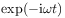. [64.1.4.2] The complex dieelectric constant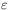is written as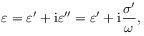(4.1)

where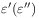are the real (imaginary) parts of, and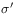is the real part of the complex conductivity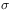. [64.1.4.3] SI units will be used. [64.1.4.4] Values forare given in multiples of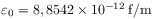, the permittivity of free space, and the units forare then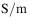. S/m. [64.1.4.5] The conductivity is written as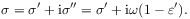(4.2)

[64.1.4.6] The relationship betweenandis also written as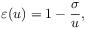(4.3)

where the notation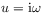has been used.

[64.1.5.1] The constituent materials are assumed to be rock forming the matrix and water filling the pore space. [64.1.5.2] Their dielectric constants are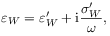(4.4)

for water, and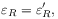(4.5)

for rock. [64.1.5.3] In calculations the values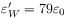and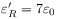will be used. [64.1.5.4] Dimensionless frequencies are introduced by setting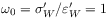for the relaxation frequency of water, and this also fixes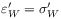.

[64.2.1.1] The average local dielectric constants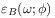and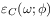at porosity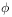are in general unknown. [64.2.1.2] Nevertheless, some general statements can be made. [64.2.1.3] The relation(4.6)

must hold for all. [64.2.1.4] It expresses the fact that the blocking geometry is nonconducting. [64.2.1.5] The following relations for the low- and high-porosity limits are also obvious: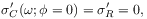(4.7a)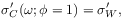(4.7b)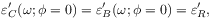(4.7c)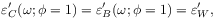(4.7d)

and they are valid for all frequencies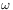.

[64.2.2.1] Finally, the local simplicity hypothesis will be cast into mathematical form by requiring that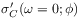can be expanded for samllas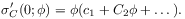(4.8)

[64.2.2.2] Correspondingly, for the blocking geometries the real dielectric constant diverges as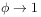, which is a thin-plate effect. [64.2.2.3] Local simplictiy is assumed to imply that the expension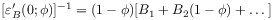(4.9)

is vaild for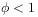. [64.2.2.4] Note that equation (4.7d) implies a discontinuity at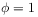.

[64.2.3.1] For the local porosity distribution, it will be assumed that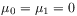in equation (2.8) and thus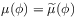. [64.2.3.2] The local percolation probability has to assume the limiting values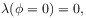(4.10a)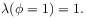(4.10b)

The first equation states that there are no conducting geometries with porosity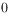, and the second says that there exist no blocking geometries at porosity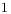.